# Introduction To Machine Learning K-Nearest Neighbors (KNN) Algorithm In Python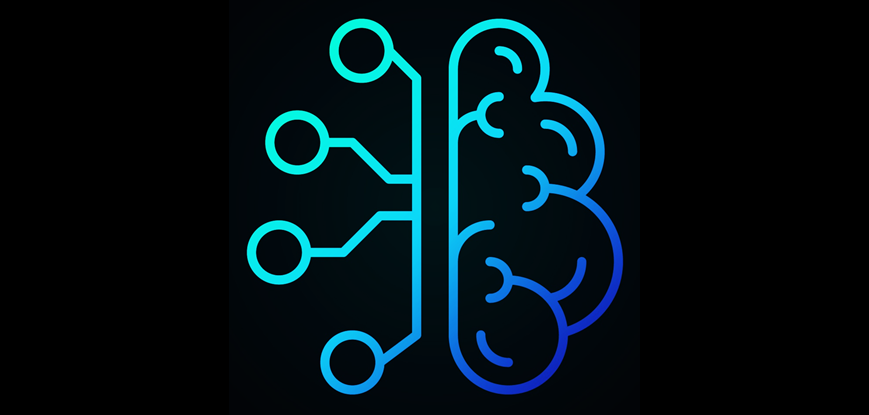Machine Learning is one of the most popular approaches in Artificial Intelligence. Over the past decade, Machine Learning has become one of the integral parts of our life. It is implemented in a task as simple as recognizing human handwriting or as complex as self-driving cars. It is also expected that in a couple of decades, the more mechanical repetitive task will be over. With the increasing amounts of data becoming available there is a good reason to believe that Machine Learning will become even more prevalent as a necessary element for technological progress. There are many key industries where ML is making a huge impact: Financial services, Delivery, Marketing and Sales, Health Care to name a few. However, here we will discuss the implementation and usage of Machine Learning in trading.

In this blog, we will give you an overview of the K-Nearest Neighbors (KNN) algorithm and understand the step by step implementation of trading strategy using K-Nearest Neighbors in Python.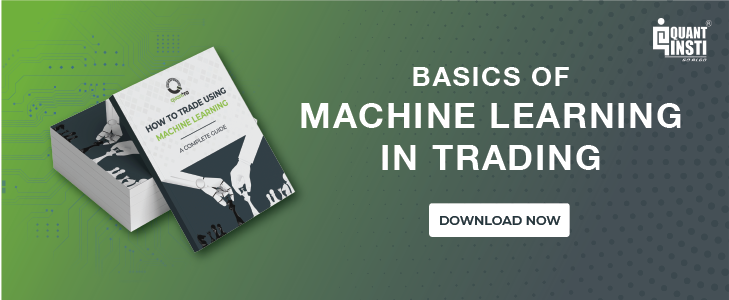K-Nearest Neighbors (KNN) is one of the simplest algorithms used in Machine Learning for regression and classification problem. KNN algorithms use data and classify new data points based on similarity measures (e.g. distance function). Classification is done by a majority vote to its neighbors. The data is assigned to the class which has the nearest neighbors. As you increase the number of nearest neighbors, the value of k, accuracy might increase.

Now, let us understand the implementation of K-Nearest Neighbors (KNN) in Python in creating a trading strategy.

### 1. Import the Libraries

We will start by importing the necessary libraries required to implement the KNN Algorithm in Python. We will import the numpy libraries for scientific calculation. Next, we will import the matplotlib.pyplot library for plotting the graph. We will import two machine learning libraries KNeighborsClassifier from sklearn.neighbors to implement the k-nearest neighbors vote and accuracyscore from sklearn.metrics for accuracy classification score. We will also import fixyahoo_finance package to fetch data from Yahoo.

###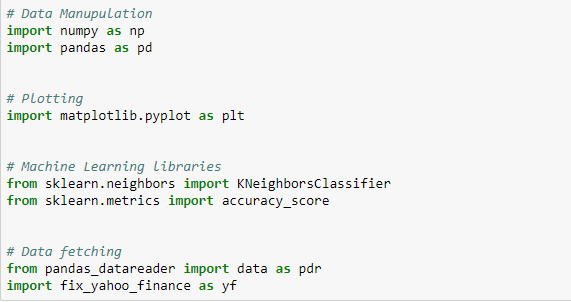2. Fetch the Data

We will fetch the S&P 500 data from yahoo finance using ‘pandas_datareader’. We store this in a data frame ‘df’. After this, we will drop all the missing values from the data using ‘dropna’ function and print the first five rows of column ‘Open’, ‘High’, ‘Low’, ‘Close’.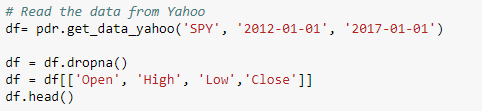Output: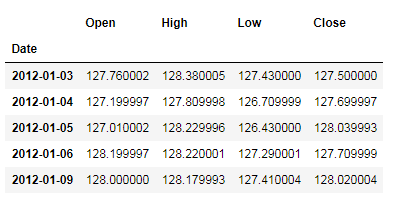### 3. Define Predictor Variable

Predictor variable, also known as an independent variable is used to determine the value of the target variable. We use ‘Open-Close’ and ‘High-Low’ as a predictor variable. We will drop the NaN values and store the predictor variables in ‘X’.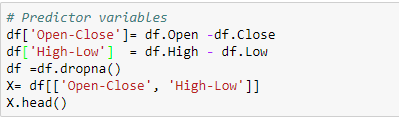Output: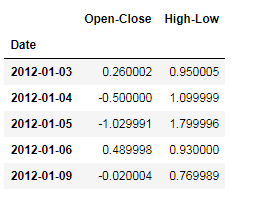### 4. Define Target Variables

The target variable, also known as the dependent variable is the variable whose values are to be predicted by predictor variables. In this, the target variable is whether S&P 500 price will close up or down on the next trading day. The logic is that if the tomorrow’s closing price is greater than today’s closing price, then we will buy the S&P 500, else we will sell the S&P 500. We will store +1 for the buy signal and -1 for the sell signal. We will store the target variable in a variable ’Y’.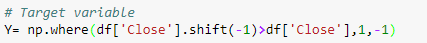### 5. Split the Dataset

Now, we will split the dataset into training dataset and test dataset. We will use 70% of our data to train and the rest 30% to test. To do this, we will create a split parameter which will divide the dataframe in a 70-30 ratio. You can change the split percentage as per choice, but it is advisable to give at least 60% data as train data for good results.

‘Xtrain’ and ‘Ytrain’ are train dataset. ‘Xtest’ and ‘Ytest’ are test dataset.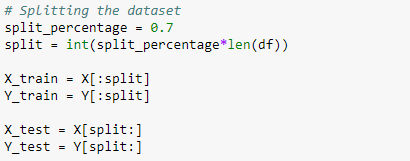### 6. Instantiate KNN Model

After splitting the dataset into training and test dataset, we will instantiate k-nearest classifier. Here we are using ‘k =15’, you may vary the value of k and notice the change in result. Next, we fit the train data by using ‘fit’ function. Then, we will calculate the train and test accuracy by using ‘accuaracy_score’ function.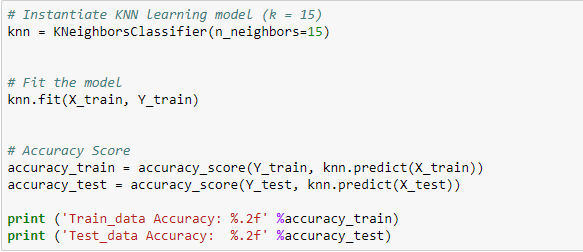Output: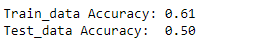Here, we see that an accuracy of 50% in a test dataset which means that 50% of the time our prediction will be correct.

### 7. Create trading strategy using the model

Our trading strategy is simply to buy or sell. We will predict the signal to buy or sell using ‘predict’ function. Then, we will calculate the cumulative S&P 500 returns for test dataset. Next, we will calculate the cumulative strategy return based on the signal predicted by the model in the test dataset. Then, we will plot the cumulative S&P 500 returns and cumulative strategy returns and visualize the performance of the KNN Algorithm.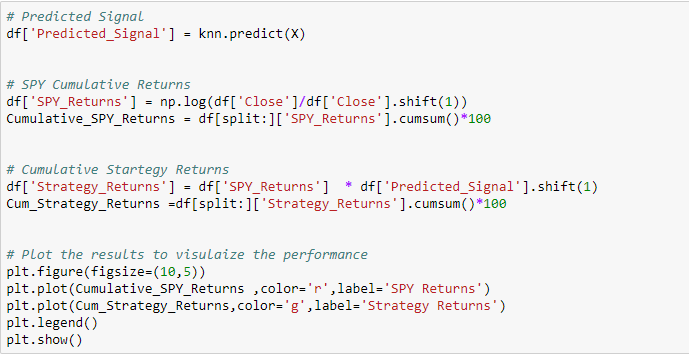Output: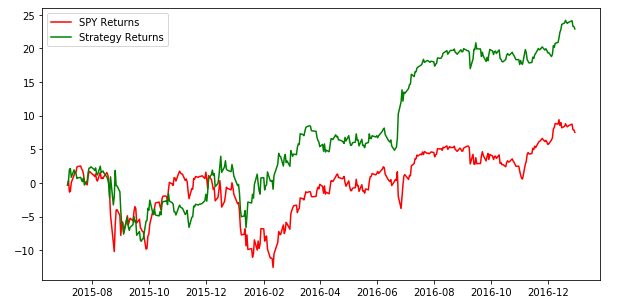This is clear from the graph that cumulative S&P 500 returns from 01-Jan-2012 to 01-Jan-2017 are around 10% and cumulative strategy returns in the same period are around 25%.

### 8. Sharpe Ratio

The Sharpe ratio is the return earned in excess of the market return per unit of volatility. First, we will calculate the standard deviation of the cumulative returns, and use it further to calculate the Sharpe ratio.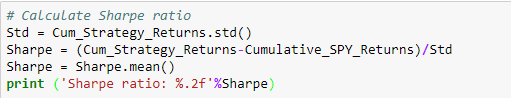Output: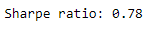The Sharpe ratio of our strategy is 0.78.

### Now, it is your turn to implement the KNN Algorithm!

You can tweak the code in the following ways.

1. You can use and try the model on the different dataset.
2. You can create your own predictor variable using different indicators that could improve the accuracy of the model.
3. You can change the value of K and play around with it.
4. You can change the trading strategy as you wish.

### Next Step

Now that you know how to implement the KNN Algorithm in Python, you can start to learn how logistic regression works in machine learning and how you can implement the same to predict stock price movement in Python. Click here to read now.

Update

We have noticed that some users are facing challenges while downloading the market data from Yahoo and Google Finance platforms. In case you are looking for an alternative source for market data, you can use Quandl for the same.

Disclaimer: All investments and trading in the stock market involve risk. Any decisions to place trades in the financial markets, including trading in stock or options or other financial instruments is a personal decision that should only be made after thorough research, including a personal risk and financial assessment and the engagement of professional assistance to the extent you believe necessary. The trading strategies or related information mentioned in this article is for informational purposes only.